Smartick is an advanced online program that teaches kids math and coding in only 15 min. a day

Sep16

# Factorization: What Is It and How Is It Done?

In the post for this week, we will take a look at factorization. What is it? Why do we use it? How is it done? These are some of the questions we will answer in this post.

### What is factorization?

Factors of a number are numbers that divide evenly into another number. Factorization writes a number as the product of smaller numbers.

For example, let’s factor the number 12:

12 = 6 x 2 or

12 = 3 x 4 or

12 = 2 x 2 x 3

### Why do we use factorization?

It can be used for many things, like helping perform arithmetic operations.

For example 15 x 8
Factor 15 and 8:

15 = 3 x 5

8= 2 x 4

Now we group the factors so that it is easier for us to multiply.

(2 x 5) x (3 x 4) = 10 x 12 = 120

Another way to use factorization is to find the least common multiple and greatest common factor. But for this, factorization has to be done using prime numbers. This is called prime factorization.

### How do we find the prime factorization of a number?

The most common way is to divide the number by its prime divisors until only the number 1 is left.

For example, let’s complete the prime factorization of the number 24.

The number that will be factored is placed in the upper left side of a vertical line.Now we are looking for a prime factor of 24. Since 24 is an even number, we know that 2 is a factor of 24. So we write 2 next to the 24 but on the other side of the vertical line, as shown in the following image:Now we divide 24 by 2. The result is 12, and we write it below 24.

(Remember that the remainder of the division must always be zero).Now we have to find a prime factor of number 12. It is also an even number so we know that 2 is a factor of 12. We write a 2 across the line from 12.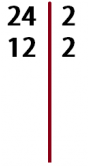We divide 12 by 2 and the result is 6. We write it below the 12.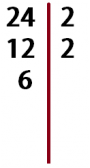We look for a prime factor of 6 and, once again, it is 2. We write it on the right.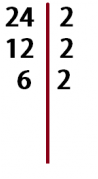We divide 6 by 2 and we get 3. The result is written below 6.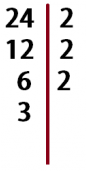Now we look for a prime factor of 3. Since 3 is a prime number, its only prime factor is itself.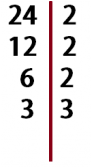Divide 3 by 3 and the result is 1. We write the result below 3 and have finished the prime factorization of the number 24.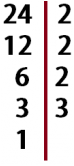The prime factors of the number 24 are found to the right of the vertical line.

24 = 2 x 2 x 2 x 3

Remember that in Smartick you can learn to use the factorization and all subjects of mathematics for children between 4 and 14 years. Sign up now for a free trial.